# Kinematics Principles: Help and Review Chapter Exam

Exam Instructions:

Choose your answers to the questions and click 'Next' to see the next set of questions. You can skip questions if you would like and come back to them later with the yellow "Go To First Skipped Question" button. When you have completed the practice exam, a green submit button will appear. Click it to see your results. Good luck!

### Page 1

#### Question 2 2. This graph is broken up into 5 sections, labeled A-E. On this graph, what section(s) represents positive acceleration?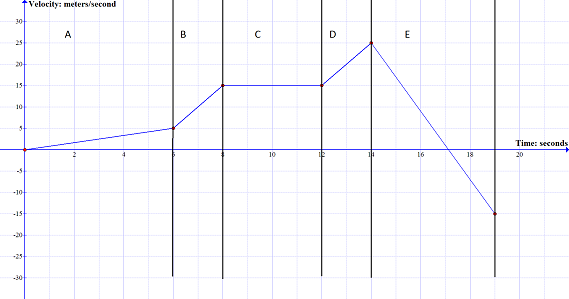### Page 2

#### Question 7 7. On this graph, what is the acceleration between 10 seconds and 14 seconds?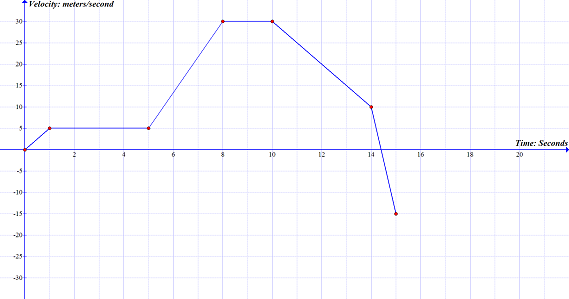### Page 3

#### Question 11 11. Given this Position vs. Time graph, which of the following Velocity vs. Time graphs represents the same type of motion?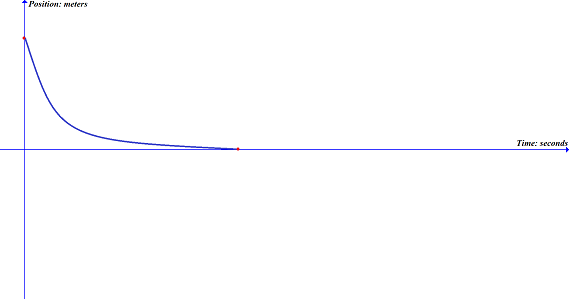#### Question 12 12. This graph represents how fast you jogged over a specific period of time. If you traveled 3 meters in 1 second, using the graph, what label should be at position A?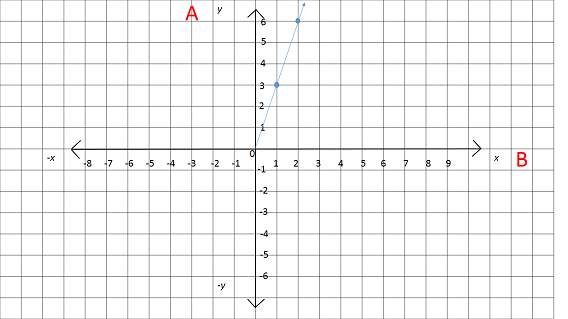#### Question 14 14. Using the graph, calculate the displacement for the section labeled A.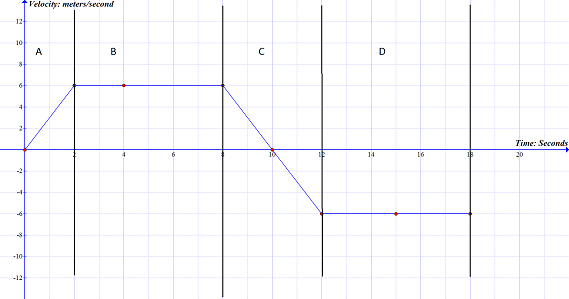#### Question 15 15. Looking at this graph, what assumption can you make about the displacement of the object over the time period indicated by the letter A?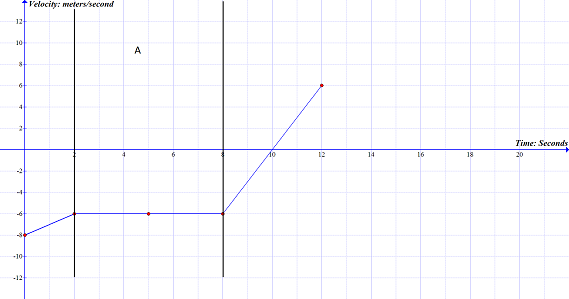### Page 4

#### Question 16 16. This graph is broken up into 5 sections, labeled A-E. On this graph, what section(s) represents no acceleration?#### Question 18 18. This graph represents an object that is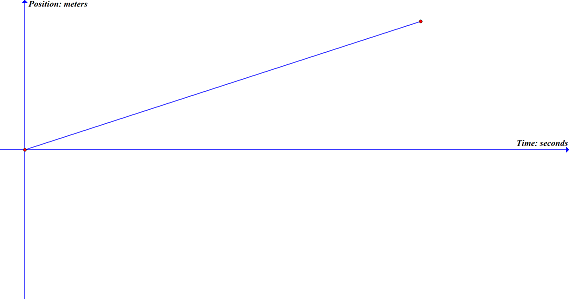#### Question 20 20. Given this Velocity vs. Time graph, which of these Position vs. Time graphs represents the same kind of motion?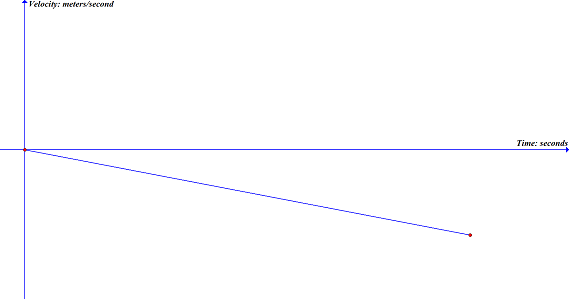### Page 6

#### Question 26 26. Calculate the displacement for some object in the diagram that starts at Start and ends at Finish.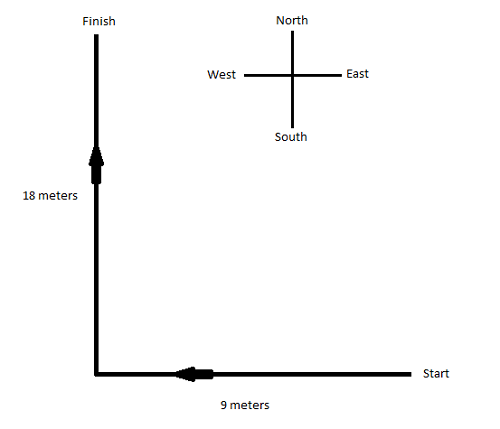#### Question 27 27. On this graph, what is the change in velocity between 5 seconds and 8 seconds?#### Question 28 28. On this graph, what is the acceleration between 1 seconds and 5 seconds?#### Kinematics Principles: Help and Review Chapter Exam Instructions

Choose your answers to the questions and click 'Next' to see the next set of questions. You can skip questions if you would like and come back to them later with the yellow "Go To First Skipped Question" button. When you have completed the practice exam, a green submit button will appear. Click it to see your results. Good luck!

Support